## 第一章 绪论

• 传码率$R_B$：单位时间内传输码元（可以是二进制、多进制）的个数，单位 Baud/s

• 传信率$R_b$：单位时间内传输的比特总数，单位 bit/s或bps

• 关系：$R_b =R_B \log_2M$ 其中 M 为进制数

• $\eta=\frac{R_B}{B} ~~(Baud/Hz)$
• $\eta_b=\frac{R_b}{B} ~~(bit/s/Hz)$

• 码元差错率：$P_e=\frac{错误码元数}{传输的总码元数}$
• 信息差错率：$P_e=\frac{错误比特数}{传输的总比特数}$

## 第二章 随机信号与噪声分析

### 随机过程X(t)的数字特征

• 数学期望：$E[X(t)]=\int_{-\infty}^{+\infty}x f(x, t)dx$

• 方差：$D[X(t)] = E\{[X(t)-a(t)]^2\}$ 其中a(t)为数学期望

• 自相关函数（重要）：$R(t_1, t_2)=E[X(t_1)X(t_2)]$

• 协方差函数：$B(t_1, t_2)=E\{[X(t_1)-a(t_1)][X(t_2)-a(t_2)]\}$

$B(t_1, t_2)=R(t_1, t_2)-a(t_1)a(t_2)$ (重要)

### 平稳随机过程

• 均值与时间t无关，为常数$a$
• 自相关函数只与时间间隔有关，为$R(\tau)$

• 一维情况：的概率密度函数与时间t无关

• $E[X(t)]=0$
• $R_X(t_1,t_2)=\frac{A^2}{2}\cos\omega \tau$，其中$\tau=t_2-t_1$

【平稳随机过程的各态历经性】：

• 时间均值：$\overline{a}=\lim_{T\to\infty}\frac{1}{T}\int_{-T/2}^{T/2} x(t)dt$
• 时间相关函数：$\overline{R(\tau)}= \lim_{T \to \infty} \frac{1}{T} \int_{-T/2}^{T/2} x(t)x(t+\tau)dt$

• $a=E[X(t)]=\overline{a}$
• $R(\tau)=\overline{R(\tau)}$

【自相关函数的性质】：

• $R(\tau)$ 为偶函数
• $R(0)=E[X^2(t)]=\sigma^2 + E^2(\infty)$ 图中的$\sigma_X^2$ 即方差，$m_X^2$$E^2(\infty)$
• $R(0)$为平均功率，$\sigma^2$为交流功率，$E^2(\infty)$ 为直流功率【功率谱密度函数$P_X(\omega)$

• 确定信号：$P_x(\omega)=\lim_{T\to \infty} \frac{|X_T(\omega)|^2}{T}$

• 随机信号：$P_X(\omega)= E[P_x(\omega)] = \lim_{T\to \infty} \frac{E[|X_T(\omega)|^2]}{T}$

• $P_X(\omega)$ 是确定函数，偶函数，非负函数

• 随机过程的平均功率=$R(0)=\frac{1}{2\pi}\int_{-\infty}^{+\infty} P_X(\omega) d\omega$

## 第四章 模拟调制系统

### 幅度调制

• 普通调幅AM

$s_{AM}(t)=[A_0+m(t)]\cos \omega_ct$ 其中m(t)为输入的调制信号，输出$s_{AM}(t)$ 为【调幅信号】，所以经过调制的信号都可称为 【已调信号】

调幅度：$\frac{\max(m(t))}{A_0}$ 调幅度小于等于 1 时可以采用包络检波

• 双边带调幅DSB

$s_{DSB}=m(t)\cdot \cos(\omega_ct)$

• 单边带调制（调制结果分为上边带、下边带两种）

• 上边带：$s_{USB}=\frac{1}{2}m(t)\cos(\omega_ct)-\frac{1}{2}\hat{m}(t)\sin(\omega_ct)$

• 下边带：$s_{LSB}=\frac{1}{2}m(t)\cos(\omega_ct)+\frac{1}{2}\hat{m}(t)\sin(\omega_ct)$

注：$\hat{m}(t)$$m(t)$ 的希尔伯特变换，即 m(t) 的正频率部分相位减去$\frac{\pi}{2}$• $P_{AM}=载波功率+边带功率=P_c+P_s=\frac{A_0^2}{2}+\frac{P_m}{2}$
• $P_{DSB}=P_s=\frac{P_m}{2}$
• $P_{SSB}=\frac{1}{2}P_s=\frac{1}{4}P_m$

• AM解调：

• 相干解调：$m_o(t)\approx \frac{1}{2}[m(t)+A_0]\overset{隔直}{\rightarrow} \frac{1}{2}m(t)$
• 包络检波：$m_o(t)\approx m(t)+A_0\overset{隔直}{\rightarrow} m(t)$
• DSB解调：

相干：$m_o(t)=\frac{1}{2}m(t)$ 输出功率：$P_o=\frac{1}{4}\overline{m^2(t)}=\frac{1}{4}P_m$

• SSB解调：

相干：$m_o(t)=\frac{1}{4}m(t)$$P_o=\frac{1}{16}\overline{m^2(t)}=\frac{1}{16}P_m$

• $G_{AM}\le 1$ (包络检波、且为大信噪比时)，单音调制，且调制度=100%时，$G_{AM}=2/3$
• $G_{DSB}=2$
• $G_{SSB}=1$

### 角度调制

• 调相：$\varphi(t)=K_P m(t)$
• 调频：$\frac{d\varphi(t)}{dt}=K_F m(t)$

• 窄带调频时，$D<<1, B_{NBFM}=2f_m$
• 宽带调频时，$B_{FM}=2(D+1)f_m$
• D为调频指数，$D=\Delta f_m/f_m$$\Delta f_m=K_F|m(t)|_{\max}$ 为最大频偏，单音调制时用$m_f$代替 D

• 非相干解调$m_o(t)=K_dK_Fm(t)$
• 相干解调：$m_o(t)=\frac{1}{2}A_0K_Fm(t)$

## 第五章 数字基带传输系统

### 各种编码

• 单极性不归零码

• 双极性不归零码

• 单极性归零码

• 双极性归零码

• AMI码（传号反转码，1码交替用正、负电平表示，0码用低电平表示）

• HDB3码（解决AMI码中连0长串问题）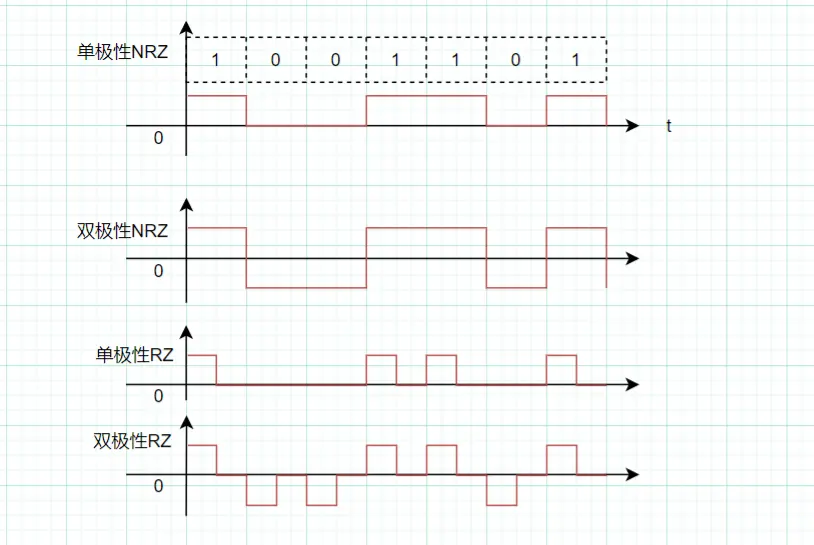### 数字基带传输

$P_s(f) = P_u(f)+P_v(f)\\ = f_bP(1-P)|G_1(f)-G_2(f)|^2+\sum_{m=-\infty}^{+\infty} f_b^2|PG_1(mf_b)+(1-P)G_2(mf_b)|^2\delta(f-mf_b)$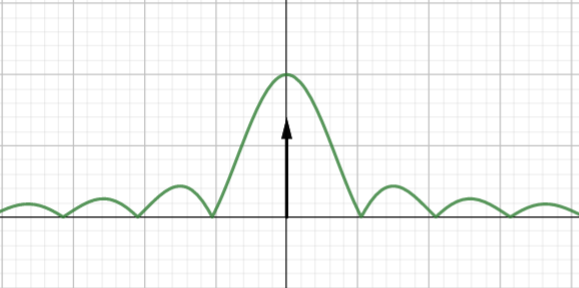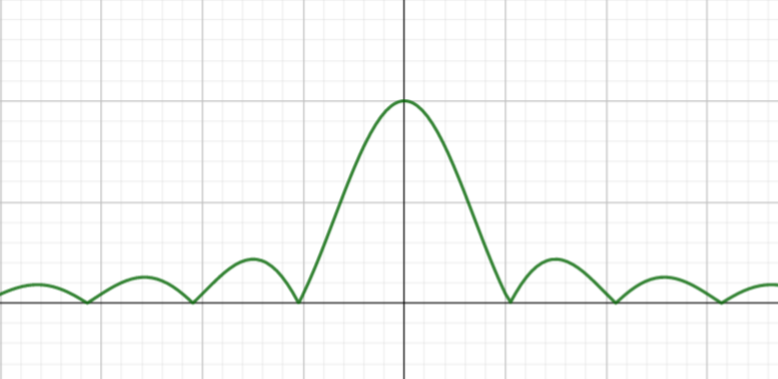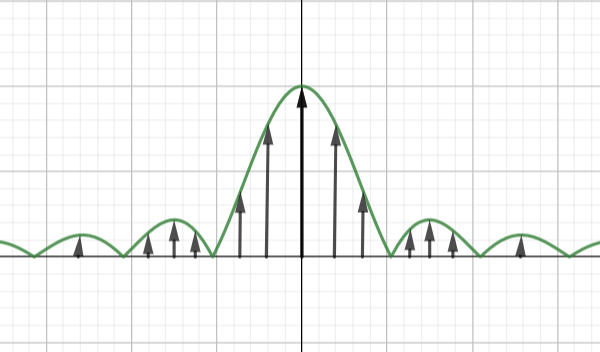### 无码间干扰

• 双极性、等概率发送0，1：$P_e=\frac{1}{2}erfc(\frac{A}{\sqrt{2}\sigma_n})$
• 单极性、等概率发送0，1：$P_e=\frac{1}{2}erfc(\frac{A}{2\sqrt{2}\sigma_n})$

### 部分响应系统

1. 预编码：$b_k=a_k\oplus b_{k-1}$
2. 相关编码（消除误码传播）：$c_k=b_k+b_{k-1}$
3. 译码：$a_k=b_k\oplus b_{k-1}=[c_k]_{\mod{2}}$

### 均衡器

$y_k=\sum_{i=-N}^N C_ix_{k-i}$

## 第六章 数字信号频带传输

### 带宽

• $B_{2ASK}=2R_B$
• $B_{2FSK}=|f_1-f_2|+2R_B$
• $B_{2DPSK}=B_{2PSK}=2R_B$

### 误码率

• 2ASK:$\frac{1}{2}erfc(\sqrt{\frac{r}{4}})$, r>>1时，$\frac{1}{\sqrt{\pi r}} e^{-r/4}$
• 2FSK:$\frac{1}{2} erfc(\sqrt{\frac{r}{2}})$, r>>1时，$\frac{1}{\sqrt{2\pi r}} e^{-r/2}$
• 2PSK:$\frac{1}{2}erfc(\sqrt{r})$, r>>1时，$\frac{1}{2\sqrt{\pi r}} e^{-r}$
• 2DPSK:$erfc(\sqrt{r})$, r>>1时，$\frac{1}{\sqrt{\pi r}} e^{-r}$
• 4PSK:$erfc(\sqrt{r}\sin\frac{\pi}{4})$
• 4DPSK:$erfc(\sqrt{2r}\sin\frac{\pi}{8})$

• 2DPSK（差分相干解调）:$\frac{1}{2}e^{-r}$
• 2FSK（包络检波）:$\frac{1}{2}e^{-r/2}$
• 2ASK（包络检波）:$\frac{1}{2}e^{-r/4}$

## 第七章 现代数字调制技术

QAM：带宽、频率利用率记一下即可

## 第八章 模拟信号的数字传输

### 采样定理

$f_s=2f_m$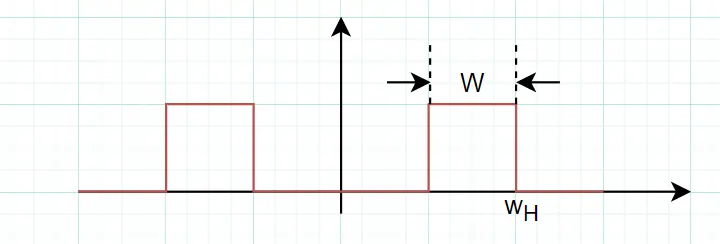$\omega_s=2W(1+\frac{M}{N})$

• $N= floor(\omega_H/W)$，也就是$\omega_H/W$的整数部分
• $M=\frac{\omega_H}{W}-N$, 且$0\le M < 1$

### 量化

• 最小量化间隔：$\Delta A=\frac{b-a}{M}$，最大值b，最小值a，M个量化区间
• 最大量化误差：$(\Delta q)_{\max}=\Delta A/2$
• 量化信噪比：$\frac{S_o}{N_o}=M^2$

A律压缩曲线：

$y=\begin{cases} \frac{Ax}{1+\ln A} & 0

• 大信噪比时：$\frac{S_o}{N_o}=2^{2N_b},N_b\ge \log_2M$
• 小信噪比时：$\frac{S_o}{N_o}=\frac{1}{4P_e}$, Pe为误码率

### PCM编码

• 符号位$b_7$：1-正，0-负；1268为正数，则$b_7=1$

• 段落码$b_6b_5b_4$：根据下表判断该取哪个值，比如，抽样值为567，则$512\le567<1024$，则$b_6b_5b_4=110$。抽样值为1268>1024，则$b_6b_5b_4=111$

抽样值$b_6b_5b_4$
1024111
512110
256101
128100
64011
32010
16001
0000
• 段内码$b_3b_2b_1b_0$：将对应区间分成均匀分为16份，比如抽样值为567，所在区间为[512, 1024)，分成16份，每份大小为(1024-512)/16=32；题中给的抽样值 1268>1024，因此1268所在的区间为[1024, 2048)，每份大小ΔA=(2048-1024)/16=64，则

0000对应1024

0001对应1024+64*1

0010对应1024+64*2

1111对应1024+64*15

• 因为 1024+64*3=1216 < 1268 < 1024+64*4=1280，因此1268对应的$b_3b_2b_1b_0=0011$

• 综上，得到最终编码为 11110011

• 误差计算

• 采用起始电平为译码电平时，则实际上11110011对应1216，因此 误差=1268-1216=52
• 通常采用中间电平为译码电平，即在原来的基础上加 ΔA/2，则1110011对应译码后应该为 1216+64/2=1248，因此最终【误差】=1268-1248=20

### 时分复用TDM

• $f_s=2f_m$,$T_s=\frac{1}{2f_m}$
• 每个0、1信号占用时间：$T_b=\frac{T_s}{N_b n}$, n代表几路信号进行复用
• $R_B=1/T_b$
• 无码间干扰带宽：$B=R_B/2$
• 主瓣带宽：$B=1/\tau$，τ表示码元宽度

## 第九章 差错控制编码

### 基本概念

• $d_0\ge e+1$，可以检错e个码
• $d_0\ge 2t+1$，可以纠错t个码
• $d_0\ge t+e+1且e\ge t$，可以检查出e个错，同时纠正其中t个错

### 线性分组码

• 其编码效率为$k/n$

• 监督矩阵$H_0=[P~~~I_r]$，其中$I_r$代表单位矩阵，阶数为r，H0共n列，r行。（注：H0是典型化的矩阵，不是典型化需要进行【矩阵初等变化】，才能得到典型化的矩阵）

• 生成矩阵$G_0=[I_k~~~P^T]$，其中$I_k$代表单位矩阵，阶数为k，G0共n列，k行。（注：G0是典型化的矩阵，不是典型化需要进行【矩阵初等变化】，才能得到典型化的矩阵）

【译码方式】

$|S^T|-1=j$，则 R 中从又往左数（0开始）第j个码发生错误，记得在回答时纠正过来

### 循环码

• g(x)最高次项为$x^{n-k}$，常数项为1
• 一般题目会给出许用码组，在许用的码组中找出一个码字，其中$a_{n-k}$左边的码均为0，第n-k个码$a_{n-k}$为1，最右边的码$a_0$为1，然后根据这个码的$a_1,a_2,\cdots,a_{n-k-1}$来判断$g_1,g_2,\cdots,g_{n-k-1}$是多少。

• 即证$x^n-1$ 能被$g(x)$ 整除

• 先求监督多项式$r(x)\equiv x^{n-k}m(x)，模g(x)$

• $A(x)=m(x)x^{n-k} + r(x)$

m(x)为信息码多项式

• 利用所有码多项式均能被 g(x) 整除的性质

## 第十章 数字信号的最佳接收

### 最大信噪比准则与匹配滤波器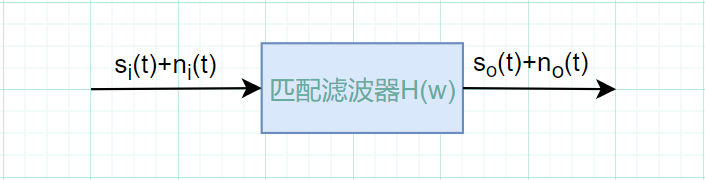• 输出噪声功率谱$P_{no}(\omega)=\frac{n_o}{2}|H(\omega)|^2$,$n_0/2$是输入高斯白噪声的功率谱密度函数$P_{ni}$
• 输出噪声总功率$N_o=\frac{1}{2\pi}\int_{-\infty}^{+\infty}P_{no}(\omega)d\omega$
• 输入信号功率$E_i=\frac{1}{2\pi}\int_{-\infty}^{+\infty}|S_i(\omega)|^2d\omega$
• 最大输出信噪比$r_{omax}=\frac{E_i}{n_0/2}$

• $h(t)=Ks_i(t_0-t)$，通常取$K=1, t_0=T_b$
• $H(\omega)=KS_i^*(\omega)e^{-j\omega t_0}$### 最小误码率准则与相关接收机

$P(s_1)P(x|s_1)>P(s_2)P(x|s_2)$ 则判断为s1, 否则为s2，相等时无法判断，误码率最大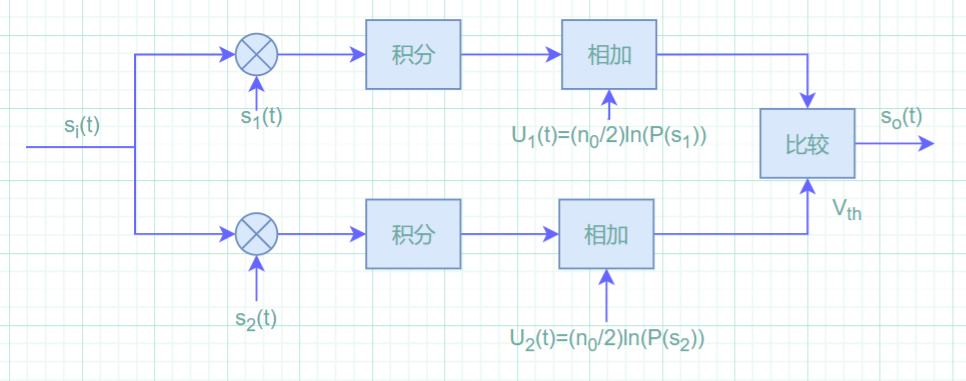### 最佳接收机误码率

$P_e=\frac{1}{2}erfc(\sqrt{\frac{E_b(1-\rho)}{2n_0}})$

• 当使用 双极性码，PSK发送时，ρ=-1
• ρ=1时，Pe=0.5，误码率最大

## 第十一章 同步原理

### 载波同步

• 插入导频法
• 直接提取法
• 平方变换法（平方环法）
• 同相正交环法

### 位同步

• 外同步法
• NRZ码：在$\omega_b$处插入导频
• RZ码，在$\omega_b/2$ 处插入导频，然后2倍频即可得到$\omega_b$
• 自同步法
• 模拟锁相环法
• 带限整流法
• 数字锁相环